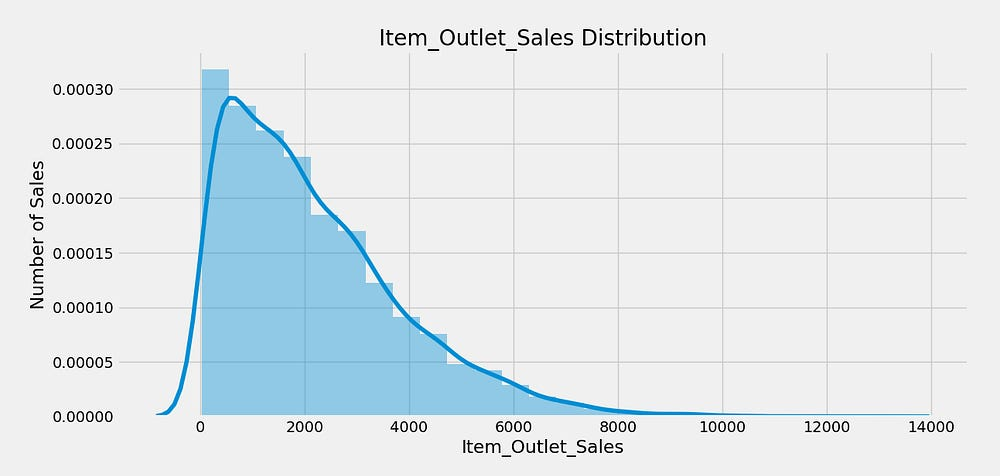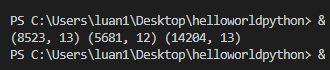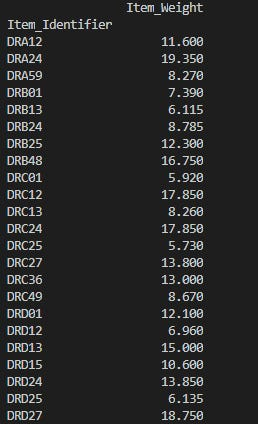# Project 1 : Bigmart Sale Prediction

Hi guys, I’m Luan. I come from Viet Nam. This article is the first one on my medium. I’m quite bad at English, so I wanna write more to improve English skill. This is also the first project when I start reaching with Machine Learning and Deep Learning. If having any mistake, I hope all people can condone to me. Thank everyone a lot.

This project I refer to Diogo Menezes Borges and Ryan. The following links :

Now we go to be specific into this project.

Introduction about data :

According to the provided information, Big Mart is a big supermarket chain. The data have been collected from in 2013, including 1559 products across 10 stores in different cities. They are provided to challenge all Data Scientist to make this corporation sales predictor model to ensure success of their business.

Process makes this model corresponding to the following steps :
1. Exploring Data.
2. Data Pre-processing.
3. Feature Engineering.
4. Creating Model.
5. Evaluation.

1. Exploring data.
Everyone can explicitly see this part via this link : https://medium.com/diogo-menezes-borges/project-1-bigmart-sale-prediction-fdc04f07dc1e . Here I only present summarily.
1.1 Distribution of the target variableItem_Outlet_Sales Distribution

We can see that our target variable is skewed to the right. So we will concentrate on that.

1.2 Distribution of the variable Item_Fat_Content

See that the variable Item_Fat_Content includes five values. So we should correct into two values LF and Regular.

1.3 Distribution of the variable `Item_Type`

We see that there are sixteen different types. We need to think of the way to reduce this

1.4 Distribution of the variable `Outlet_Location_Type`

1.5 Distribution of the variable `Outlet_Type`

Supermarket type2, Grocery Store, Supermarket type3 have low expression in the distribution. Maybe we should gather them into the single category.

1.2. Bivariate Analysis

Once again, I please emphasize that this article I have referred to https://medium.com/diogo-menezes-borges/project-1-bigmart-sale-prediction-fdc04f07dc1e
http://unsupervisedlearning.co.uk/2017/11/22/project-3-big-mart-sales-prediction-part-2/

Firstly we will analyze numerical variables

1.2.1 `Item_Weight` and `Item_Outlet_Sales` analysis

We see that the variable Item_weight have low correlation with the variable Item_Outlet_Sales.

1.2.2 `Item_Visibility` and `Item_Outlet_Sales` analysis

Maybe, we think that Visibility of items will have high correlation to Sales of Items. However, seeing at the above picture, we see that items have low Visibility which still have high sales. Even, Items with Visibility equal 0 still sell very well.

1.2.3 Impact of Outlet_Identifier on Item_Outlet_Sales

1.2.4 `Outlet_Establishment_Year` and `Item_Outlet_Sales` analysis

To seem to have no significant meaning between Outlet_Establishment_Year and Item_Outlet_Sales
1.2.5 Impact of `Outlet_Size` on `Item_Outlet_Sales`

The variable Outlet_Size probably don’t have high correlation with our target variable. Most stores have size “Medium” but still the “High” and “Small” stores which are clearly in an inferior number can beat or even come close to their numbers.

1.2.6 Impact of `Outlet_Type` on `Item_Outlet_Sales`

From this analysis possibly it would be a good idea to creat a new feature that shows the sales ratio according to the store size.

Other variables Everyone can see at link that I already give on the head of this article.

2. Data Pre-Processing

After exploring data, we can give out some conclusion :
`Item_Visibility` does not have a high positive correlation as expected
As well, there are no big variations in the sales due to the`Item_Type` .
– If we look at variable `Item_Identifer` , we can see different groups of letters per each product such as ‘FD’ (Food), ‘DR’(Drinks) and ‘NC’ (Non-Consumable). From this we can create a new variable.
`Item_Fat_Content` has vale “low fat” writen in different manners.
– For `Item_Type` we try to create a new feature that does not have 16 unique values.
`Outlet_Establishment_Year` besides being a hidden category, its values vary from 1985 to 2009 . It must be converted to how old the store is to better see the impact on sales.

2. 1. Looking for missing values

Firstly, we need to combine train data and test data to avoid the trouble of repeting twice the same code

`# Join Train and Test Dataset`
`#Create source column to later separate the data easilytrain['source']='train'test['source']='test'`
`data = pd.concat([train,test], ignore_index = True)print(train.shape, test.shape, data.shape)`Result

Next, we remove NaN value . Numpy’s `NaN`values are good because Pandas is able to recognize this object and count it.

We see that 39,9 % Item_Outlet_Sales is NaN value. So we will change them into mean value.

2.2. Imputing Missing Values

2.2.1. Imputing the `mean` for `Item_Weight` missing values

data.pivot_table() (refer to this link ). this method allows us to create a table with all the identifiers and their respective weight. Since this method ignores all `NaN` values and the same item exists in more than one store, for those missing the weight we can retrieve from this table the `mean()` weight of all products with the same `Item_Identifier`.

`#aggfunc is mean by default! Ignores NaN by default`
`item_avg_weight = data.pivot_table(values='Item_Weight', index='Item_Identifier')`
`print(item_avg_weight)`Mean()
`data[:][data[‘Item_Identifier’] == ‘DRI11’]`
`def impute_weight(cols):    Weight = cols    Identifier = colsif pd.isnull(Weight):return item_avg_weight['Item_Weight'][item_avg_weight.index == Identifier]else:return Weight`
`print ('Orignal #missing: %d'%sum(data['Item_Weight'].isnull()))`
`data['Item_Weight'] = data[['Item_Weight','Item_Identifier']].apply(impute_weight,axis=1).astype(float)`
`print ('Final #missing: %d'%sum(data['Item_Weight'].isnull()))`

Running above code, we have the following result :

NaN values have changed equal mean value.

2.2.2. Imputing `Outlet_Size` missing values with the `mode`

For this example, we will apply the same logic. In this case, instead of using the default code`aggfunc = mean()` for the `pivot_table()`we will use the `mode`

`def impute_size_mode(cols):    Size = cols    Type = colsif pd.isnull(Size):return outlet_size_mode.loc['Outlet_Size'][outlet_size_mode.columns == Type]else:return Size`
`print ('Orignal #missing: %d'%sum(data['Outlet_Size'].isnull()))data['Outlet_Size'] = data[['Outlet_Size','Outlet_Type']].apply(impute_size_mode,axis=1)print ('Final #missing: %d'%sum(data['Outlet_Size'].isnull()))`

Running above code, we have the following result :

3. Feature Engineering

3.1 `Item_Visibility` minimum value is 0

In our data, seeing that visibility have the value 0, which makes no sense. since every product must be visible to all clients. Let’s consider it as missing value and impute it with mean visibility of that product.

`def impute_visibility_mean(cols):    visibility = cols    item = colsif visibility == 0:return visibility_item_avg['Item_Visibility'][visibility_item_avg.index == item]else:return visibility`
`print ('Original #zeros: %d'%sum(data['Item_Visibility'] == 0))data['Item_Visibility'] =`
`data[['Item_Visibility','Item_Identifier']].apply(impute_visibility_mean,axis=1).astype(float)`
`print ('Final #zeros: %d'%sum(data['Item_Visibility'] == 0))`

As a result :

3.2 Determine the years of operation of a store

We concern working time instead of Establishment time. Hence we will handle them thank to the following code :

#Remember the data is from 2013
data[‘Outlet_Years’] = 2013 — data[‘Outlet_Establishment_Year’]
data[‘Outlet_Years’].describe()

3.3 Create a broad category of `Item_Type`

Segment Item_Type into 3 category including “FD” (Food), “DR” (Drinks) or “NC” (Non-Consumables)

`#Get the first two characters of ID:data['Item_Type_Combined'] = data['Item_Identifier'].apply(lambda x: x[0:2])`
`#Rename them to more intuitive categories:data['Item_Type_Combined'] = data['Item_Type_Combined'].map({'FD':'Food',                                                      'NC':'Non-Consumable',                                                            'DR':'Drinks'})`
`data['Item_Type_Combined'].value_counts()`

As a result :

3.4 Modify categories of `Item_Fat_Content`

As analyzed in above part, we need to correct the variable Item_Fat_Content into 2 category( Low_Fat and Regular ).

`#Change categories of low fat:print('Original Categories:')print(data['Item_Fat_Content'].value_counts())`
`print('\nModified Categories:')data['Item_Fat_Content'] = data['Item_Fat_Content'].replace({'LF':'Low Fat',                                                      'reg':'Regular','low fat':'Low Fat'})`
`print(data['Item_Fat_Content'].value_counts())`

We also saw that there were some non-consumables as well and a fat-content should not be specified for them. So we can also create a separate category for such kind of observations.

As a result :

4. Feature Transformations [taken from ]

4.1. Categorical Variables — One Hot Encoding

Because `scikit-learn` only accepts numerical variables, we need to convert all categories of nominal variables into numeric types. Let’s start with turning all categorical variables into numerical values using `LabelEncoder()` (Encode labels with value between 0 and n_classes-1). After that, we can use `get_dummies` to generate dummy variables from these numerical categorical variables

`#Import library:from sklearn.preprocessing import LabelEncoderle = LabelEncoder()`
`#New variable for outletdata['Outlet'] = le.fit_transform(data['Outlet_Identifier'])`
`var_mod = ['Item_Fat_Content','Outlet_Location_Type','Outlet_Size','Item_Type_Combined','Outlet_Type','Outlet']`
`for i in var_mod:    data[i] = le.fit_transform(data[i])`

One-Hot-Coding refers to creating dummy variables, one for each category of a categorical variable. For example, the `Item_Fat_Content` has 3 categories — `LowFat`,`Regular`,`Non-Edible`. One hot coding will remove this variable and generate 3 new variables. Each will have binary numbers — 0 (if the category is not present) and 1(if category is present). This can be done using `get_dummies` function of Pandas.

`#Dummy Variables:data = pd.get_dummies(data, columns =['Item_Fat_Content','Outlet_Location_Type','Outlet_Size','Outlet_Type','Item_Type_Combined','Outlet'])`
`data.dtypes`

4.2 Exporting Data

After analyzing and handling data, we will separate our data into train data and test data. Running below code :

`#Drop the columns which have been converted to different types:data.drop(['Item_Type','Outlet_Establishment_Year'],axis=1,inplace=True)`
`#Divide into test and train:train = data.loc[data['source']=="train"]test = data.loc[data['source']=="test"]`
`#Drop unnecessary columns:test.drop(['Item_Outlet_Sales','source'],axis=1,inplace=True)train.drop(['source'],axis=1,inplace=True)`
`#Export files as modified versions:train.to_csv("data/train_modified.csv",index=False)test.to_csv("data/test_modified.csv",index=False)`

5. Model Building

train_df = pd.read_csv(‘data/train_modified.csv’)
test_df = pd.read_csv(‘data/test_modified.csv’)

Refer to

5.1 Linear Regression

`mean_sales = train_df['Item_Outlet_Sales'].mean()`
`baseline_submission = pd.DataFrame({`
`'Item_Identifier':test_df['Item_Identifier'],`
`'Outlet_Identifier':test_df['Outlet_Identifier'],`
`'Item_Outlet_Sales': mean_sales`
`},columns=['Item_Identifier','Outlet_Identifier','Item_Outlet_Sales'])`
`print(baseline_submission)`
`from sklearn.linear_model import LinearRegression`
`lr = LinearRegression(normalize=True)`
`X_train = train_df.drop(['Item_Outlet_Sales','Item_Identifier','Outlet_Identifier'],axis=1)`
`Y_train = train_df['Item_Outlet_Sales']`
`X_test = test_df.drop(['Item_Identifier','Outlet_Identifier'],axis=1).copy()`
`lr.fit(X_train, Y_train)`
`lr_pred = lr.predict(X_test)`
`lr_accuracy = round(lr.score(X_train,Y_train) * 100,2)`
`print('sai so la %.4g' %lr_accuracy)`
`#submission`
`linear_submission = pd.DataFrame({`
`'Item_Identifier':test_df['Item_Identifier'],`
`'Outlet_Identifier':test_df['Outlet_Identifier'],`
`'Item_Outlet_Sales': lr_pred`
`},columns=['Item_Identifier','Outlet_Identifier','Item_Outlet_Sales'])`
`linear_submission.to_csv('linear_algo.csv',index=False)`

5.2 #Decision tree

`from sklearn.tree import DecisionTreeRegressor`
`tree = DecisionTreeRegressor(max_depth=15,min_samples_leaf=100)`
`tree.fit(X_train,Y_train)`
`tree_pred = tree.predict(X_test)`
`tree_accuracy = round(tree.score(X_train,Y_train)*100,2)`
`print('sai so dicision la : %.4g'%tree_accuracy)`
`tree_submission = pd.DataFrame({`
`'Item_Identifier':test_df['Item_Identifier'],`
`'Outlet_Identifier':test_df['Outlet_Identifier'],`
`'Item_Outlet_Sales': tree_pred`
`},columns=['Item_Identifier','Outlet_Identifier','Item_Outlet_Sales'])`
`tree_submission.to_csv('tree_algo.csv',index=False)`

5.3 #randomForest

`#randomForest`
`from sklearn.ensemble import RandomForestRegressor`
`rf = RandomForestRegressor(n_estimators=400,max_depth=6, min_samples_leaf=100,n_jobs=4)`
`rf.fit(X_train,Y_train)`
`rf_pred = rf.predict(X_test)`
`rf_accuracy = round(rf.score(X_train,Y_train)*100,2)`
`print('sai so randomforest la : %.4g' %rf_accuracy)`

5.4 Result :

Conclusion : Dicision tree have the best result with 61.58 %.

Source: Deep Learning on Medium